# Electronics and Communication Engineering - Exam Questions Papers

16.

A plane wave having the electric field component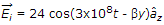V/m and travelling in free space in incident normally on a lossless medium with m = m0 and e = 9e0 which occupies the region y ≥ 0. The reflected magnetic field component is given by

 A.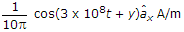B.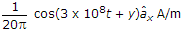C.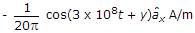D.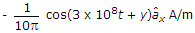Explanation: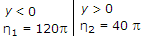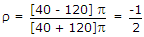r = - Ve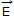will change direction and M will not change its direction

Direction of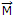for incident field is in x, so direction ofin the reflected field is also is in x.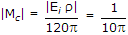.

17.

For the circuit shown in the following figure, transistors M1 and M2 are identical NMOS transistors. Assume that M2 is in saturation and the output is unloaded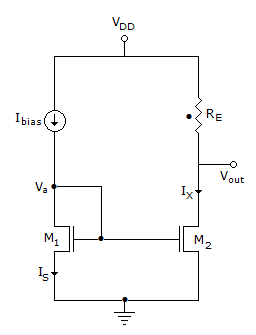The current Ix is related to Ibias as

 A. Ix = Ibias + Is B. Ix = Ibias C. Ix = Ibias - Is D.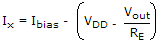Explanation:

Given circuit is current mirror IG = 0
Ix = Ibias .

18.

I1(s) and I2(s) are the Laplace transforms of i1(t) and i2(t) respectively. The equations for the circuit shown in above figure when switch S is closed at t = 0; are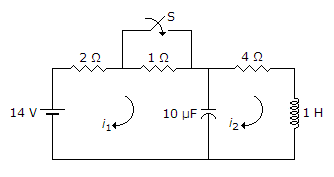A.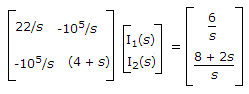B.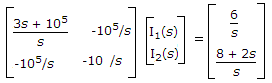C.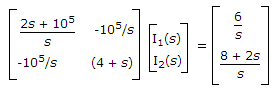D.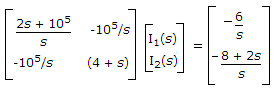Explanation: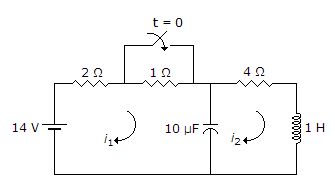For t < 0, steady state is reached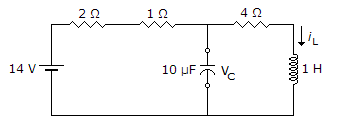iL = 2A L = 2

VC = 8 V

Using Laplace transformation for t = 0+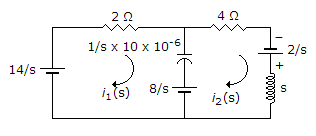Apply KVL to mesh 1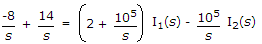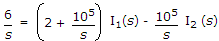Apply KVL to mesh 2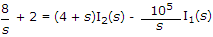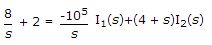.

19.

The residues of a complex function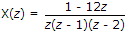at its poles are

 A.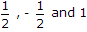B.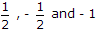C.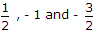D.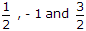Explanation: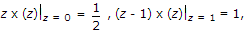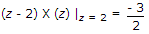.

20.

A signal with ± 10 V ranged and 1 KHz band width is being digitized using a sample and hold circuit and a 10 bit quantizer. The quantization step is :

 A. 1 x 10-9 V B. 5 x 10-9 V C. 2.5 x 10-9 V D. 0.5 x 10-9 V

Quantization step =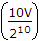.